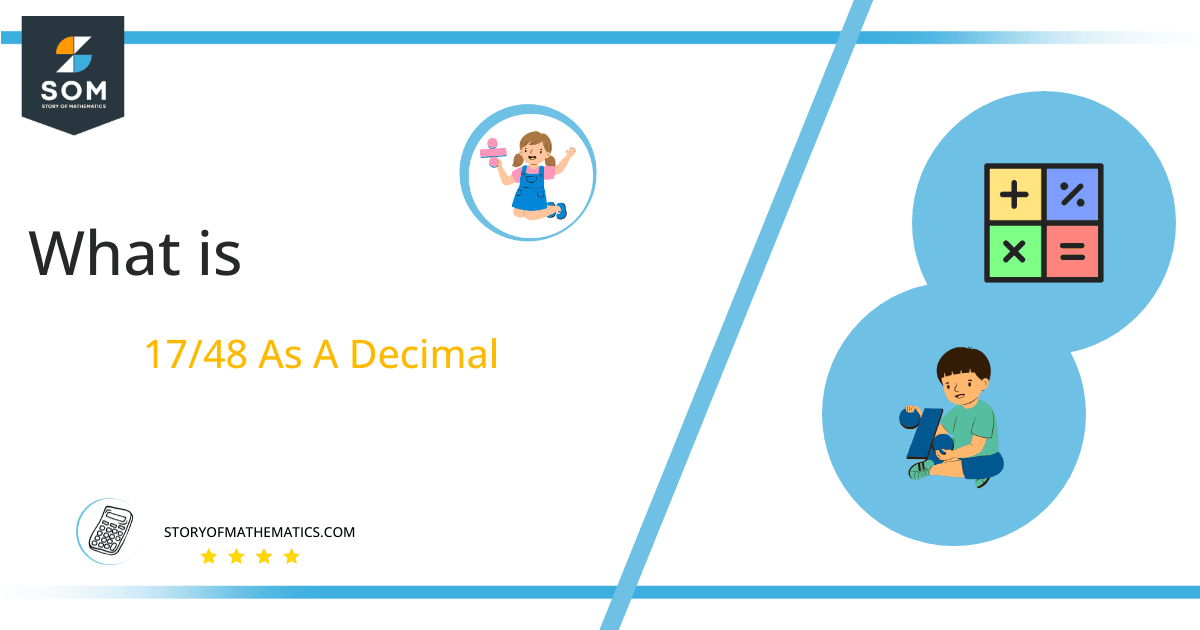# What Is 17/48 as a Decimal + Solution With Free Steps

The fraction 17/48 as a decimal is equal to 0.354166666.

A Fraction can be represented in p/q form, where p and q are referred to as the Numerator and Denominator, respectively. Fractions involve Division, and division is one of the toughest mathematical operations among all operators. But we can make it simple by using the method discussed later.

Here, we are more interested in the division types that result in a Decimal value, as this can be expressed as a Fraction. We see fractions as a way of showing two numbers having the operation of Division between them that result in a value that lies between two Integers.Now, we introduce the method used to solve said fraction to decimal conversion, called Long Division, which we will discuss in detail moving forward. So, let’s go through the Solution of fraction 17/48.

## Solution

First, we convert the fraction components, i.e., the numerator and the denominator, and transform them into the division constituents, i.e., the Dividend and the Divisor, respectively.

This can be done as follows:

Dividend = 17

Divisor = 48

Now, we introduce the most important quantity in our division process: the Quotient. The value represents the Solution to our division and can be expressed as having the following relationship with the Division constituents:

Quotient = Dividend $\div$ Divisor = 17 $\div$ 48

This is when we go through the Long Division solution to our problem.Figure 1

## 17/48 Long Division Method

We start solving a problem using the Long Division Method by first taking apart the division’s components and comparing them. As we have 17 and 48, we can see how 17 is Smaller than 48, and to solve this division, we require that 17 be Bigger than 48.

This is done by multiplying the dividend by 10 and checking whether it is bigger than the divisor or not. If so, we calculate the Multiple of the divisor closest to the dividend and subtract it from the Dividend. This produces the Remainder, which we then use as the dividend later.

Now, we begin solving for our dividend 17, which after getting multiplied by 10 becomes 170.

We take this 170 and divide it by 48; this can be done as follows:

170 $\div$ 48 $\approx$ 3

Where:

48 x 3 = 144

This will lead to the generation of a Remainder equal to 170 – 144 = 26. Now this means we have to repeat the process by Converting the 26 into 260 and solving for that:

260 $\div$ 48 $\approx$ 5

Where:

48 x 5 = 240

This, therefore, produces another Remainder which is equal to 260 – 240 = 20. Now we must solve this problem to Third Decimal Place for accuracy, so we repeat the process with dividend 200.

200 $\div$ 48 $\approx$ 4

Where:

48 x 4 = 192

Finally, we have a Quotient generated after combining the three pieces of it as 0.354=z, with a Remainder equal to 8.Images/mathematical drawings are created with GeoGebra.